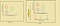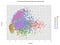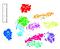# TSNE: T-Distributed Stochastic Neighborhood Embedding (State of the art)

TSNE is considered as state of the art in the area of Dimensionality Reduction (specifically for the visualization of very high dimensional data). Although there are many techniques available to reduce high dimensional data (e.g. PCA), TSNE is considered one of the best techniques available, which was the new area of the research and published the official paper in 2008. There is a beautiful website by the researcher Laurens van der Maaten himself about TSNE details.

Here in this blog, I will cover below points
1. Limitation of PCA
2. How TSNE works (Geometrically)
3. A good example of TSNE to follow
4. Sample Python code to apply TSNE
5. Drawbacks of TSNE

# Limitation of PCA

The way PCA works is that it tries to maximize the variance towards the principal component(s) and the other components (having low variance) are declared as noise points. So PCA tries to maintain the global structure of the data.

Here in the above image, we have two features (f1 & f2) along with you can see that there are two clusters of points (A & B) in both the sides of the principal component 1. So if we try to reduce 2 dimensions into 1 dimension, PCA will maximize the variance and will get the principal component 1 as the final feature. The data points of the clusters will project on the principal component 1 and will superimpose on each other, so we will lose the information.

TSNE, unlike PCA, preserves the local structures (also) of the data points.

## How TSNE works (Geometrically)The concept of Neighborhood and Embedding

As it is mentioned that TSNE preserves the local structures of the data while converting from higher dimensions to lower dimensions. That's the point where the terms Neighborhood and Embedding are useful.

In the above image (left side) we can see there are three clusters in the higher dimensional space (2-D in this case). We are calling them clusters (Neighbors though) as the intra-cluster distances are very small.

What TSNE does while reducing to lower dimension (1-D in this case) is that it embeds the points into lower dimension while preserving the distances like the higher dimension space. In the image above (right side) the higher dimension points (2-D points) are projected to the lower dimension (1-D) but the intra-cluster distances are similar, but TSNE doesn’t promise to preserve the inter-cluster distance, i.e. in a higher dimension (2-D) the Red cluster is closer to blue cluster but in the lower dimension (1-D) these clusters are quite far. This is the concept of Neighborhood Embedding.Student T Distribution

The above process discussed, has a highly mathematical base to solve the optimization. In a layman’s term, TSNE uses a symmetric probability density function in both higher and lower dimension to preserve the neighborhood. Although due to the curse of dimensionality the points in higher dimensional space tend to get crowded in lower dimensional space, causing the crowding problem. To solve the crowding problem TSNE uses the Student’s T-distribution. Again how t-distribution solves the problem has rigorous mathematics behind it, but t-distribution promises that it will try best to preserve the neighborhood by means of a probability (Stochasticity). That’s why it is T-Distributed and Stochastic.

## A good example of TSNE to follow

As I said that TSNE is state of the art for visualizing high dimensional data into a low dimension. So it would be very good if we get some visualization.

So I am going to mention a nice and terrific blog which uses this state of the art technique to simplify and visualize how TSNE works and how to use TSNE effectively. This blog is a collaboration between a lot of companies and headed by Google’s Brain on Google Research.

## Sample Python code to apply TSNE

Enough of theory! Let’s try some Python coding on how to apply TSNE on a data set.

If you have Anaconda or sci-kit learn installed in your machine along with Python then it’s just plug-and-play.

First, you have to import the module of tsne

Now grab some data of your own (I would suggest taking the popular MNIST data) and if you want you can standardize them also (recommended).

Now define your model. As we want to visualize the data so we will reduce the higher dimension to 2-D data so that we can plot.

Now you are all set to reduce that high dimension data into 2-D data using TSNE.

Bam!! You have the low dimension data that you can easily visualize using either matplotlib or seaborn plot.

As a suggestion, you can use the sklearn TSNE module but it is slow on a fairly large dataset. So there is an upgraded version of TSNE (which is the same as sklearn TSNE module) called Multicore t-SNE which runs the model in parallel and very fast on a large dataset.

Here is a very beautiful blog by Dmitry Ulyanov which clearly explains how to install and use Multicore t-SNE.

As Multicore t-SNE works in a parallel fashion so while defining the model you have to pass the number of CPU cores as a parameter.

Below is both the visualization of MNIST data using PCA and TSNE. You can clearly now understand why TSNE is state of the art in visualizing higher dimensional data into a lower dimension.PCA Visualization on MNIST datasetTSNE Visualization on MNIST dataset

For the whole source code of applying TSNE on MNIST data, you can refer to this beautiful GitHub blog maintained by O'Reilly media.

## Drawbacks of TSNE

Great things always come with a cost, so as TSNE. There are a couple of limitation of TSNE

1. Crowding problem is one of the limitations of TSNE, although Student’s T Distribution helped a lot surely, but it doesn’t guarantee you to preserve all the neighborhood points in the lower dimension, it only tries it’s best to come up with the best solution to preserve local structures of the data.
2. TSNE is computationally expensive than PCA, although I have suggested using multicore t-sne, still, the multicore t-sne is fairly computationally expensive than PCA.

# Conclusion

As I have mentioned that TSNE is a very new technique (official paper published in 2008), so you can guess that a very high and advanced mathematical foundation was used to create such a masterpiece.

So in this blog, I tried to bring the concept of TSNE as simple as possible with geometric intuition and how each of the terms (T-Distribution, Stochastic, Neighborhood, Embedding) was used.

I guess it would surely help you to have a better understanding of a layman’s term how TSNE works.

Wish you a happy machine learning :)

## More from sourajit roy chowdhury

Machine learning Engineer